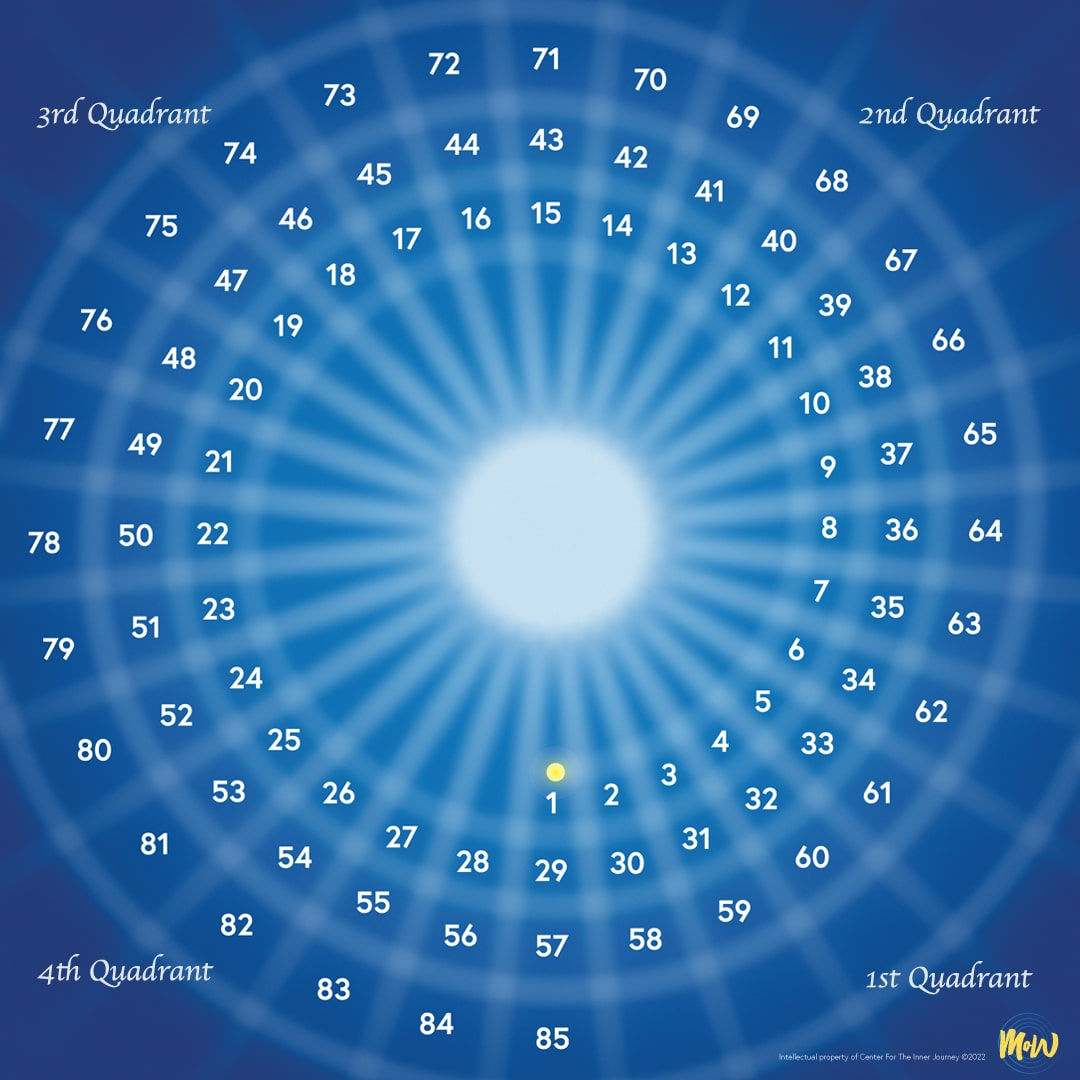On the image click on the number that represents your chronological age.Here is how it works
For instance, if your age is 60, your corresponding ages are 32 and 4. If your age is 33 your corresponding age is 5.

Next you will see how this recording is used by looking at some examples of actual MoW worksheets called The Table for Refection (TFR).

Here is how it works
For instance, if your age is 66, your corresponding ages are 38 and 10. If your age is 39 your corresponding age is 11.

Next you will see how this recording is used by looking at some examples of actual MoW worksheets called The Table for Refection (TFR).

Here is how it works
For instance, if your age is 72, your corresponding ages are 44 and 16. If your age is 46 your corresponding age is 18.

Next you will see how this recording is used by looking at some examples of actual MoW worksheets called The Table for Refection (TFR).

Here is how it works
For instance, if your age is 80, your corresponding ages are 52 and 24. If your age is 51 your corresponding age is 23.

Next you will see how this recording is used by looking at some examples of actual MoW worksheets called The Table for Refection (TFR).

Follow